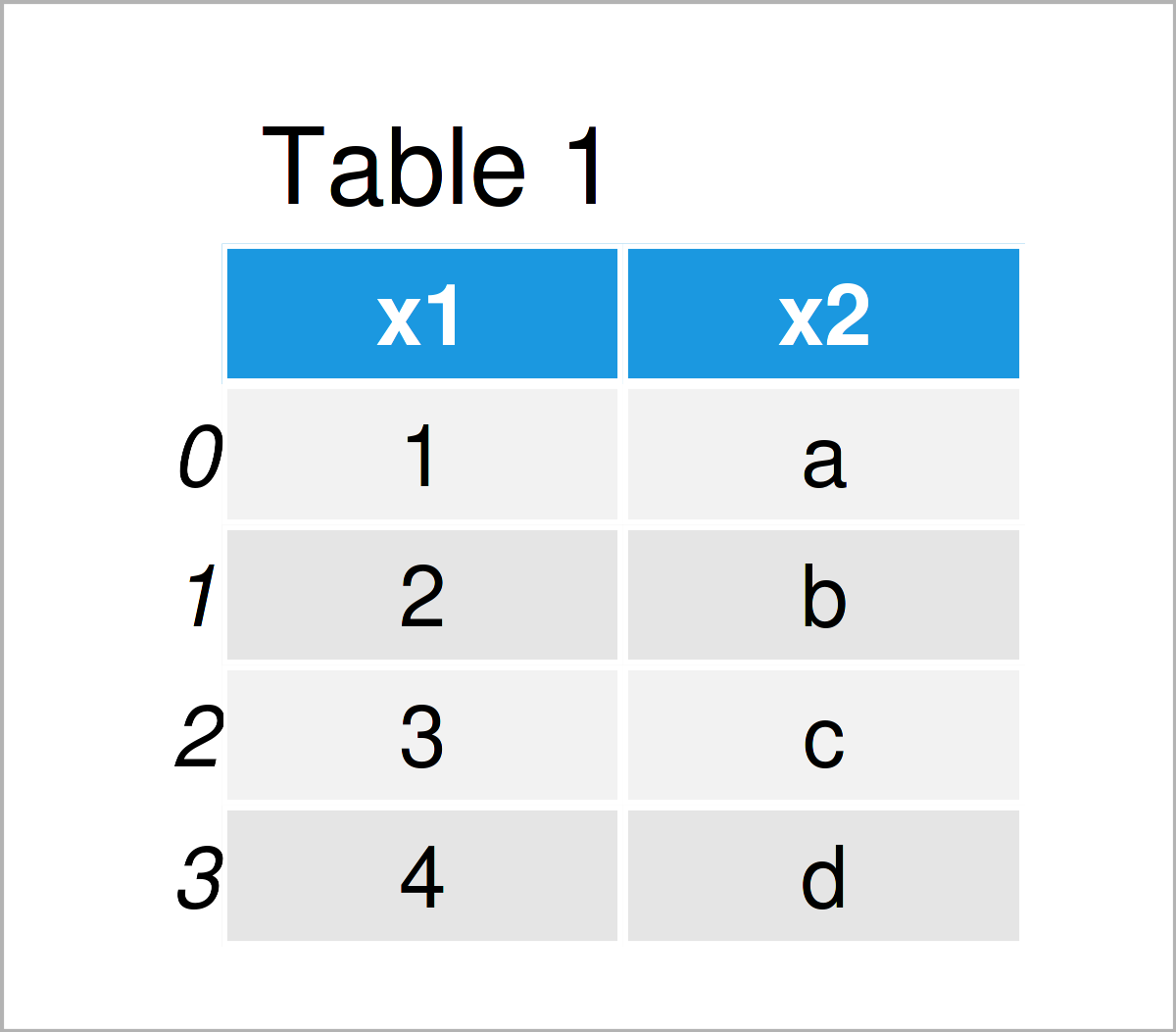# Iterate Through Rows of pandas DataFrame in Python (4 Examples)

In this post you’ll learn how to loop over the rows of a pandas DataFrame in the Python programming language.

The tutorial will consist of the following content:

Please note that looping in Python is a very controversial topic.

One major disadvantage of iterations in Python is the speed and efficiency of the code. In case you are dealing with big data sets, loops might be very slow.

On the other hand, loops might be more intuitive for some user than alternative options, and in many situations speed won’t matter.

In this tutorial, I won’t discuss the advantages and disadvantages of loops in Python in more detail.

However, in case you are interested to read more on the pros and cons of loops, I recommend having a look at this excellent thread on Stack Overflow.

Anyway, you’re here for the Python code, so let’s get straight to the tutorial…

## Example Data & Libraries

First, we need to import the pandas library:

`import pandas as pd                       # Load pandas`

We’ll also have to create some exemplifying pandas DataFrame.

```data = pd.DataFrame({'x1':range(1, 5),    # Create pandas DataFrame
'x2':['a', 'b', 'c', 'd']})
print(data)                               # Print pandas DataFrame```Table 1 shows the structure of our example data: It comprises four data points and two columns.

## Example 1: Loop Over Rows of pandas DataFrame Using iterrows() Function

The following Python code demonstrates how to use the iterrows function to iterate through the rows of a pandas DataFrame in Python.

For this task, we can use the Python syntax shown below.

In the first line of this syntax, we specify a running index (i.e. i), that we want to loop over the rows of our data set, and the name of our data set (i.e. data).

In the second line of the following code, we specify some output that should be created within the iterations of our for loop (i.e. we want to print some information about the values in each row).

Let’s do this:

```for i, row in data.iterrows():            # Use iterrows to print output
print('Index', i, '; x1 =', row['x1'], ';', 'x2 =', row['x2'])
# Index 0 ; x1 = 1 ; x2 = a
# Index 1 ; x1 = 2 ; x2 = b
# Index 2 ; x1 = 3 ; x2 = c
# Index 3 ; x1 = 4 ; x2 = d```

As you can see, the previous for loop has created one line of output for each row of our pandas DataFrame.

## Example 2: Perform Calculations by Row within for Loop

In Example 1 of this tutorial, we have used the iterrows function to print some information about our data set.

In this example, I’ll illustrate how to conduct a calculation based on the values in each row.

More precisely, we’ll multiply the value in the column x1 times five:

```for i, row in data.iterrows():            # Use iterrows to calculate by row
print(row['x1'] * 5)
# 5
# 10
# 15
# 20```

The previous output shows the values of our first column multiplied by the value five.

## Example 3: Manipulation of List within for Loop

In this example, I’ll show how to change the values stored in a list by iterating over the rows of a pandas DataFrame.

For this example, we first have to create an empty list object:

```my_list = []                              # Create empty list
print(my_list)                            # Print list
# []```

In the next step, we can modify this list by looping through the rows of our data as shown below:

```for i, row in data.iterrows():            # Use iterrows to modify list
my_list = my_list + [row['x1'] * 10]```

Let’s print our list object once again:

```print(my_list)                            # Print updated list
# [10, 20, 30, 40]```

As you can see, we have updated our list so that it now contains the values in the column x1 times ten.

## Example 4: Loop Over Rows of pandas DataFrame Using itertuples() Function

In the previous examples, we have used the iterrows function to loop through the rows of a pandas DataFrame. However, the Python programming language provides other alternatives for the iteration over DataFrame rows.

The following Python code illustrates how to use the itertuples function instead of the iterrows function. To be precise, we are reproducing the output of Example 1 by running the Python code below:

```for row in data.itertuples():             # Use itertuples instead of iterrows
print('Index', i, '; x1 =', row.x1, ';', 'x2 =', row.x2)
# Index 3 ; x1 = 1 ; x2 = a
# Index 3 ; x1 = 2 ; x2 = b
# Index 3 ; x1 = 3 ; x2 = c
# Index 3 ; x1 = 4 ; x2 = d```

Note that we had to select our column values using a dot instead of square brackets (i.e. row.x1 and row.x2).

## Video, Further Resources & Summary

Do you need more info on the topics of this tutorial? Then you could watch the following video on the Statistics Globe YouTube channel. I explain the Python programming codes of this post in the video tutorial:

Please accept YouTube cookies to play this video. By accepting you will be accessing content from YouTube, a service provided by an external third party.If you accept this notice, your choice will be saved and the page will refresh.

Furthermore, you may want to read the other tutorials on my homepage: